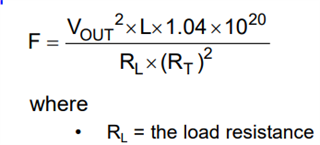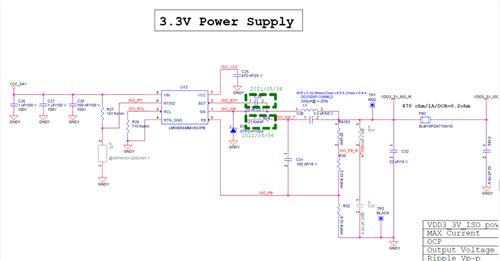If you have a related question, please click the "Ask a related question" button in the top right corner. The newly created question will be automatically linked to this question.

# LM5008A: switching frequency

Part Number: LM5008A

Dear Team

The customer use equation (1) to calculate the switching frequency.But different from the value of capture's waveform. Could you help explain the different frequency?

The spec is : Vin=54V，Vo=3.3V、Io=122mA、L=330uH、RT=191k ohm

For example, the FSW calculated by the circuit design should be about 135KHZ. If the current set by the electronic load simulation setting is 0.122A during the measurement, the Switching frequency will be about 163KHz.The switching frequency is 163kHz.Many thanks

Denny

• Hello

It looks like the converter is in CCM, so you would be using equation (2).

In any case, the equations are only approximate, since this device adjusts the Ton to

keep the frequency approximately constant with Vin.  You will also notice some variation

in the frequency with Vin.

Thanks